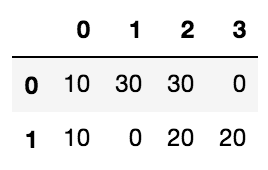# python中的filter、map、reduce、apply用法总结

• filter
• map
• reduce
• apply
• 总结

## filter

x = [1,2,3,4,5]
list(filter(lambda x:x%2==0,x)) # 找出偶数。python3.*之后filter函数返回的不再是列表而是迭代器，所以需要用list转换。
# 输出：
[2, 4]

## map

x = [1,2,3,4,5]
y = [2,3,4,5,6]
list(map(lambda x,y:(x*y)+2,x,y))
# 输出：
[4, 8, 14, 22, 32]

## reduce

from functools import reduce
y = [2,3,4,5,6]
reduce(lambda x,y: x + y,y) # 直接返回一个值

## apply

import numpy as np
a = np.random.randint(low=0,high=4,size=(2,4))
data = pd.DataFrame(a)
data.apply(lambda x:x*10)
# 输出：## 总结

1、filter和map都是python内置的函数，可以直接调用，reduce在functools模块，apply在pandas模块
2、要过滤删减序列用filter；要对多个序列做函数运算用map；在pandas里面直接调用apply，尤其是聚合对象，当然还有agg，日后补充。reduce用得少。